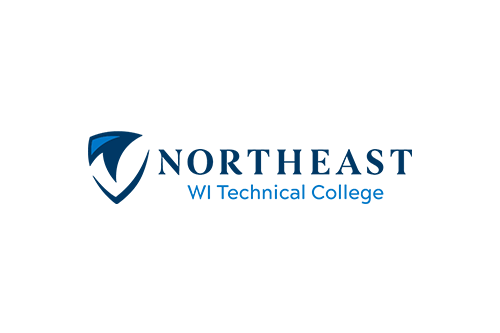# Trigonometry w Apps

Course Number: 10804196
Credits: 3.00

## Course Description

10-804-196 TRIGONOMETRY WITH APPLICATIONS ...topics include circular functions, graphing of trigonometry functions, identities, equations, trigonometric functions of angles, inverse functions, solutions of triangles complex numbers, DeMoivre's Theorem, polar coordinates, and vectors. (Prerequisite: Next Gen AAF score greater/equal to 250 AND Rdg greater/equal to 250; OR TABE A 9/10 Math greater/equal to no equiv AND Rdg greater/equal to 8.8; OR TABE 11/12 Math greater/equal to no equiv AND Rdg greater/equal to 573; OR ACT Math score greater/equal to 24 AND ACT Rdg greater/equal to 16; OR C or better in Inter Alg-contact academic advisor 920-498-5444)

• Fall
• Spring

## Course Competencies

1. Analyze trigonometric functions
2. Evaluate the trigonometric functions
3. Apply the unit circle and radian measure
4. Analyze graphs of trigonometric functions
5. Analyze inverse trigonometric functions
6. Manipulate trigonometric identities
7. Solve trigonometric equations
8. Solve oblique triangles
9. Perform vector operations
10. Perform operations with complex numbers in trigonometric form
11. Analyze the polar coordinate system